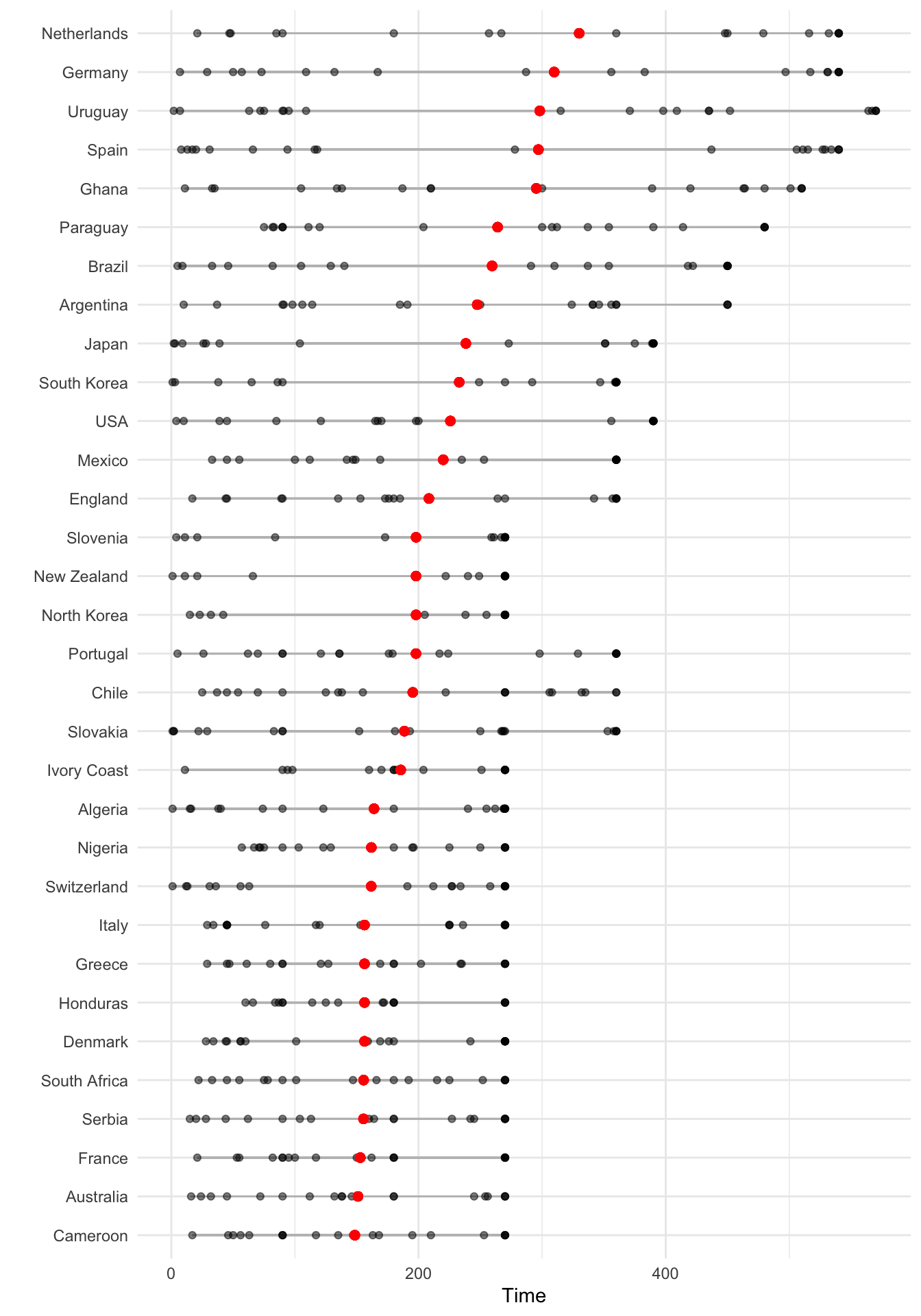## 4.2 Customizing ggplot2 Plots

With slightly more complex code, you can create very interesting and customized plots using `ggplot2`. In this section, we’ll provide an overview of some guidelines for creating good plots, based on the work of Edward Tufte and others, and show how you can customize ggplot objects to adhere to some of these guidelines. This overview will provide a framework for describing how to customize ggplot objects. We’ll end the subsection by going over scales and color specifically.

### 4.2.1 Guidelines for good plots

A number of very thoughtful books and articles have been written about creating graphics that effectively communicate information. Some of the authors we highly recommend (and from whose work we’ve pulled and aggregated the guidelines for good graphics we’ll go over) are:

• Edward Tufte (his book The Visual Display of Quantitative Information is a classic)
• Howard Wainer
• Stephen Few
• Nathan Yau

In this section, we’ll overview six guidelines for good graphics, based on the writings of these and other specialists in data display. The guidelines are:

1. Aim for high data density.
2. Use clear, meaningful labels.
3. Provide useful references.
4. Highlight interesting aspects of the data.
5. Consider using small multiples.
6. Make order meaningful.

I> While we overview some guidelines for effective plots here, this is mostly to provide a framework for showing how to customize ggplot objects. If you are interested in learning more about creating effective visualizations, you should read some of the thorough and thoughtful books written by the authors listed above. Howard Wainer’s article “How to display data badly” in The American Statistician is a particularly good place to start.

For the examples in this subsection, we’ll use `dplyr` for data cleaning and, for plotting, the packages `ggplot2`, `gridExtra`, and `ggthemes`, so you should load those packages if you plan to follow along with the examples.

``````library(dplyr)
library(ggplot2)
library(gridExtra)
library(ggthemes)``````

You can load the data for the examples in this subsection with the following code:

``````# install.packages("faraway")  ## Uncomment and run if you do not have the `faraway` package installed
library(faraway)
data(nepali)
data(worldcup)

# install.packages("dlnm")     ## Uncomment and run if you do not have the `dlnm` package installed
library(dlnm)
data(chicagoNMMAPS)
chic <- chicagoNMMAPS
chic_july <- chic %>%
filter(month == 7 & year == 1995)``````

#### 4.2.1.1 High data density

Guideline 1: Aim for high data density.

You should try to increase, as much as possible, the data to ink ratio in your graphs. This is the ratio of “ink” providing information to all ink used in the figure. In other words, if an element of the plot is redundant, take it out.

The two graphs in Figure 4.18 show the same information (“data”), but use very different amounts of ink. Each shows the number of players in each of four positions in the `worldcup` dataset. Notice how, in the plot on the right, a single dot for each category shows the same information that a whole filled bar is showing on the left. Further, the plot on the right has removed the gridded background, removing even more “ink” from the plot.

```summarise()` ungrouping output (override with `.groups` argument)``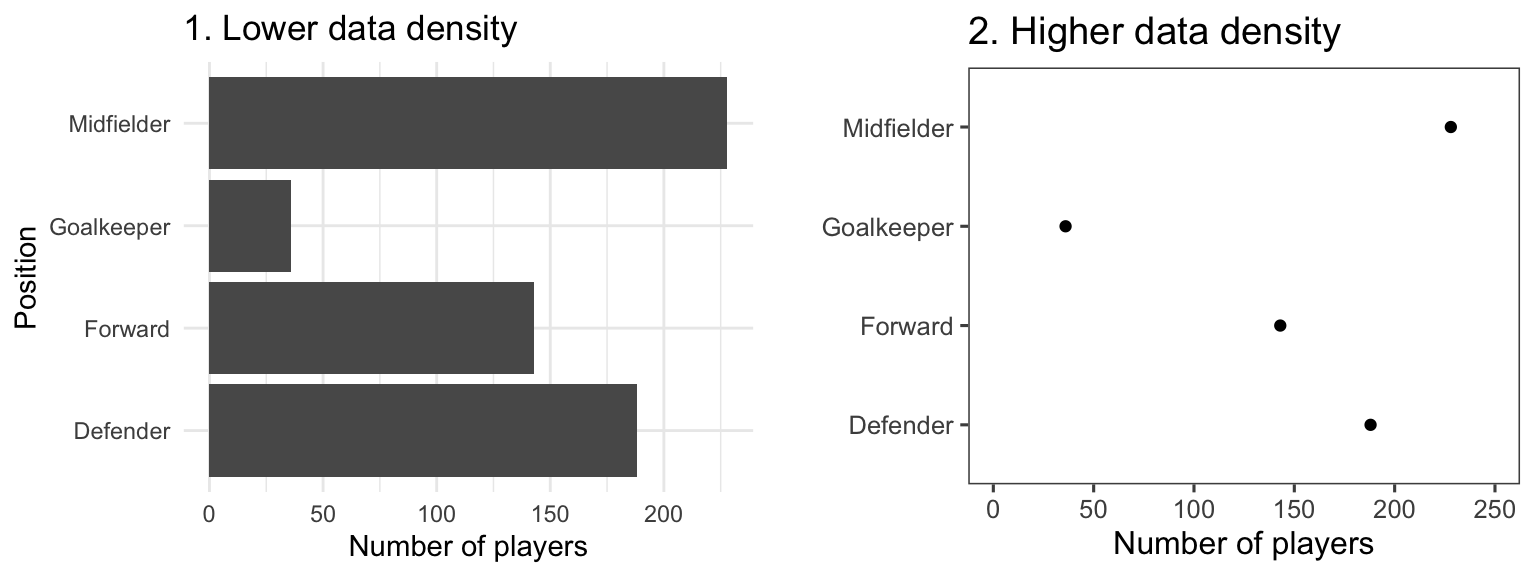Figure 4.18: Example of plots with lower (left) and higher (right) data-to-ink ratios. Each plot shows the number of players in each position in the worldcup dataset from the faraway package.

Figure 4.19 gives another example of two plots that show the same information but with very different data densities. This figure uses the `chicagoNMMAPS` data from the `dlnm` package, which includes daily mortality, weather, and air pollution data for Chicago, IL. Both plots show daily mortality counts during July 1995, when a very severe heat wave hit Chicago. Notice how many of the elements in the plot on the left, including the shading under the mortality time series and the colored background and grid lines, are unnecessary for interpreting the message from the data.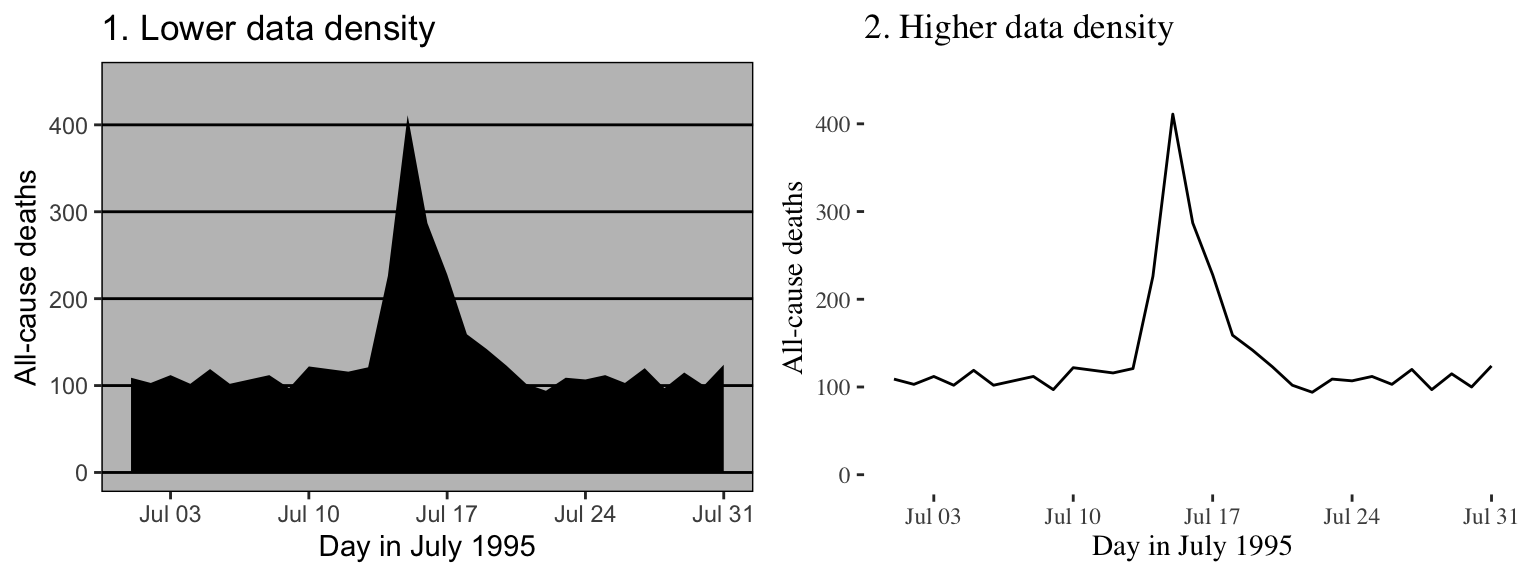Figure 4.19: Example of plots with lower (left) and higher (right) data-to-ink ratios. Each plot shows daily mortality in Chicago, IL, in July 1995 using the chicagoNMMAPS data from the dlnm package.

By increasing the data-to-ink ratio in a plot, you can help viewers see the message of the data more quickly. A cluttered plot is harder to interpret. Further, you leave room to add some of the other elements we’ll talk about, including elements to highlight interesting data and useful references. Notice how the plots on the left in Figures 4.18 and 4.19 are already cluttered and leave little room for adding extra elements, while the plots on the right of those figures have much more room for additions.

One quick way to increase data density in `ggplot2` is to change the theme for the plot, which will quickly change several elements of the plot’s appearance. There are several themes that come with `ggplot2`, including a black-and-white theme and a minimal theme. To use a theme, you can add it to a ggplot object by using a theme function like `theme_bw`. For example, to use the “classic” theme for a scatterplot using the World Cup 2010 data, you can run:

``````ggplot(worldcup, aes(x = Time, y = Shots)) +
geom_point() +
theme_classic()``````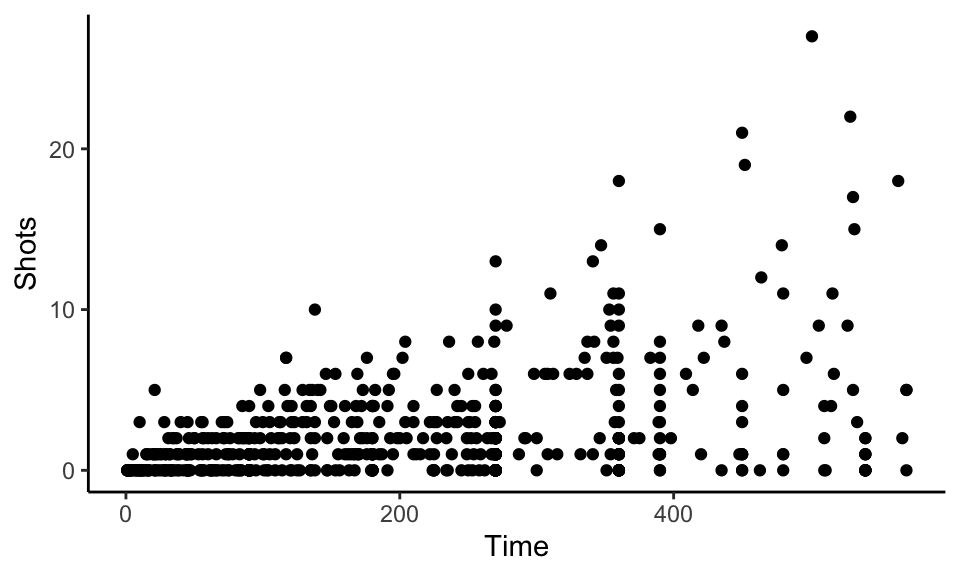Figure 4.20: Minimal theme

A number of theme functions come directly with `ggplot2`. These include:

• `theme_linedraw`
• `theme_bw`
• `theme_minimal`
• `theme_void`
• `theme_dark`
• `theme_classic`

You can find even more theme functions in packages that extend `ggplot2`. The `ggthemes` package, in particular, has some excellent additional themes. These include themes based on the graphing principles of Stephen Few (`theme_few`) and Edward Tufte (`theme_tufte`). Again, you can use one of these themes by adding it to a `ggplot` object:

``````library(ggthemes)
ggplot(worldcup, aes(x = Time, y = Shots)) +
geom_point() +
theme_tufte()``````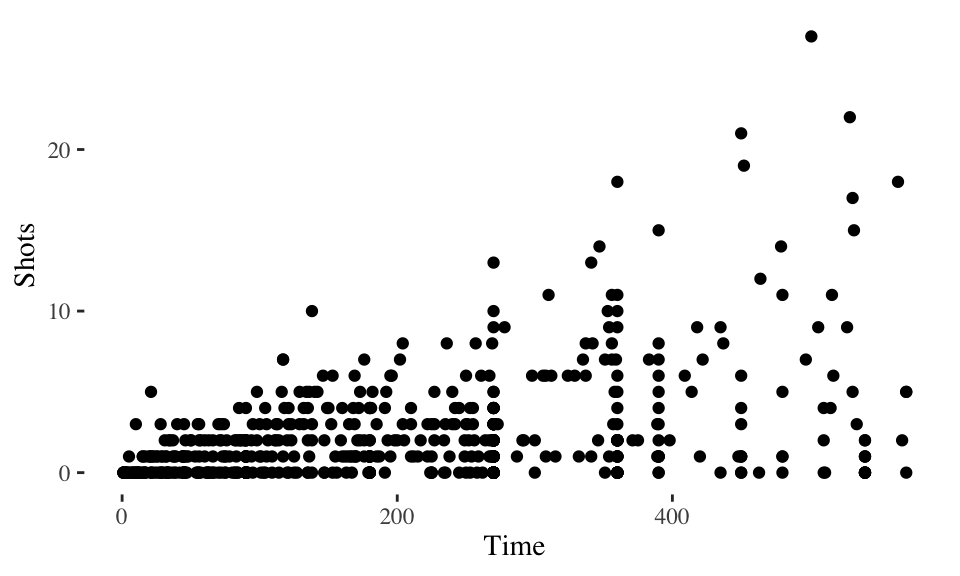Figure 4.21: Tufte theme

The plots in Figure 4.22 shows some examples of the effects of using different themes. All show the same information– a plot of daily deaths in Chicago in July 1995. The top left graph shows the graph with the default theme. The other plots show the effects of adding different themes, including the black-and-white theme that comes with `ggplot2` (top right) and various themes from the `ggthemes` package.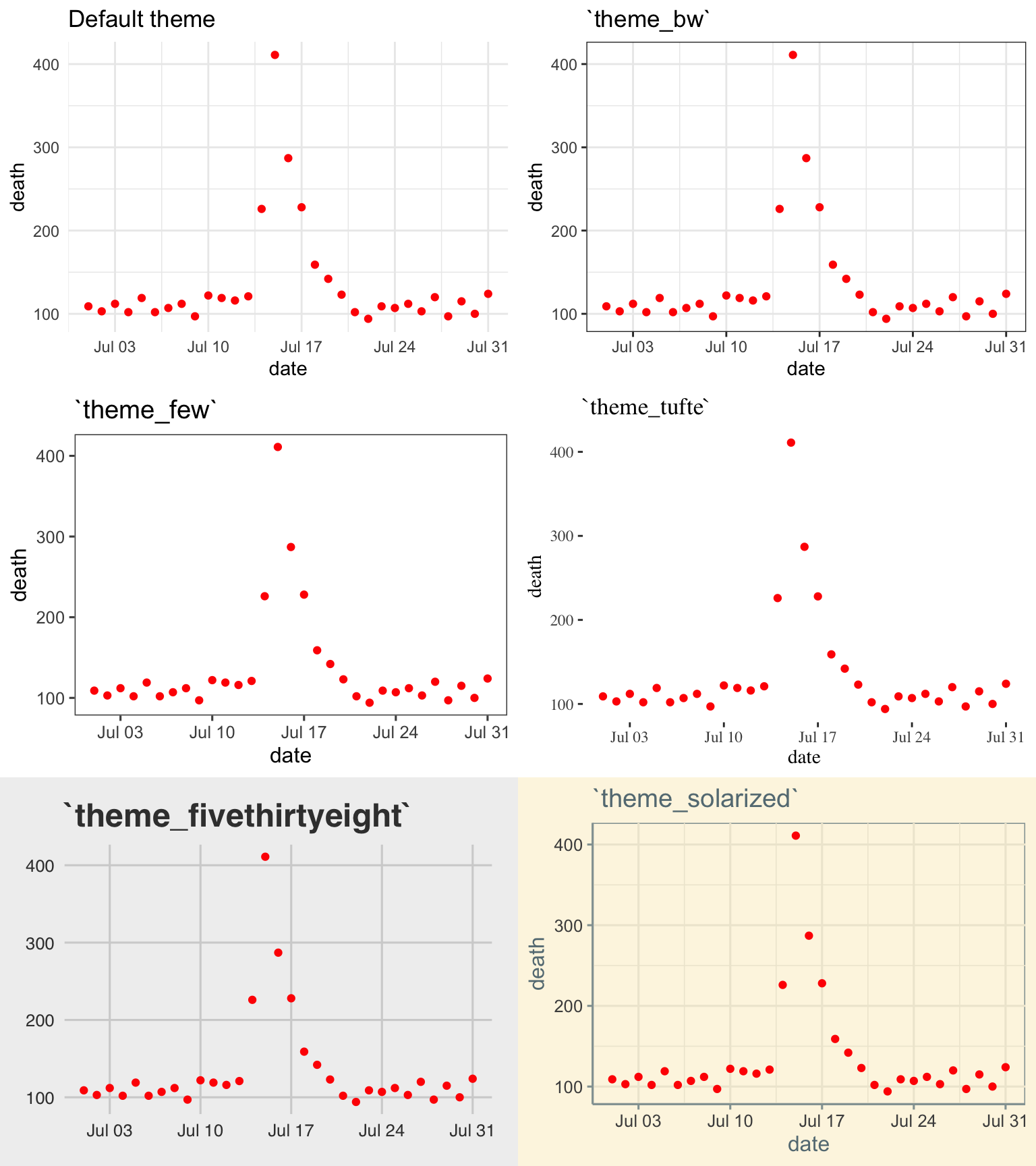Figure 4.22: Daily mortality in Chicago, IL, in July 1995. This figure gives an example of the plot using different themes.

You can see that these themes can vary sustantially in their data-to-ink ratios. Between changing themes and choosing geoms carefully, you can reduce the data-to-ink ratio in a plot substantially. For example, here is the code for the two plots from 4.19:

``````chicago_plot <- ggplot(chic_july, aes(x = date, y = death)) +
xlab("Day in July 1995") +
ylab("All-cause deaths") +
ylim(0, 450)

chicago_plot +
geom_area(fill = "black") +
theme_excel()

chicago_plot +
geom_line() +
theme_tufte() ``````

We will teach you how to make your own ggplot theme later in the course.

#### 4.2.1.2 Meaningful labels

Guideline 2: Use clear, meaningful labels.

Graphs often default to use abbreviations for axis labels and other labeling. For example, the default is for `ggplot2` plots to use column names as labels for the x- and y-axes of a scatterplot. While this is convenient for exploratory plots, it’s often not adequate for plots for presentations and papers. You’ll want to use short and easy-to-type column names in your dataframe to make coding easier (e.g., “wt”), but you should use longer and more meaningful labeling in plots and tables that others need to interpret (e.g., “Weight (kg)”).

Furthermore, text labels are often aligned in a way that makes them hard to read. For example, when plotting a categorical variable along the x-axis, it can be difficult to fit categorical labels that are long enough to be meaningful without rotating them and so making them harder to read.

Figure 4.23 gives an example of the same information (number of players in the World Cup data set by position) shown with labels that are harder to read and interpret (left) versus with clear, meaningful labels (right). Notice how the graph on the left is using abbreviations for the categorical variable (“DF” for “Defense”), abbreviations for axis labels (“Pos” for “Position” and “count” for “Number of players”), and has the player position labels in a vertical alignment. On the right graph, we have made the graph easier to quickly read and interpret by spelling out all labels and switching the x- and y-axes, so that there’s room to fully spell out each position while still keeping the alignment horizontal, so the reader doesn’t have to turn the page (or his head) to read the values.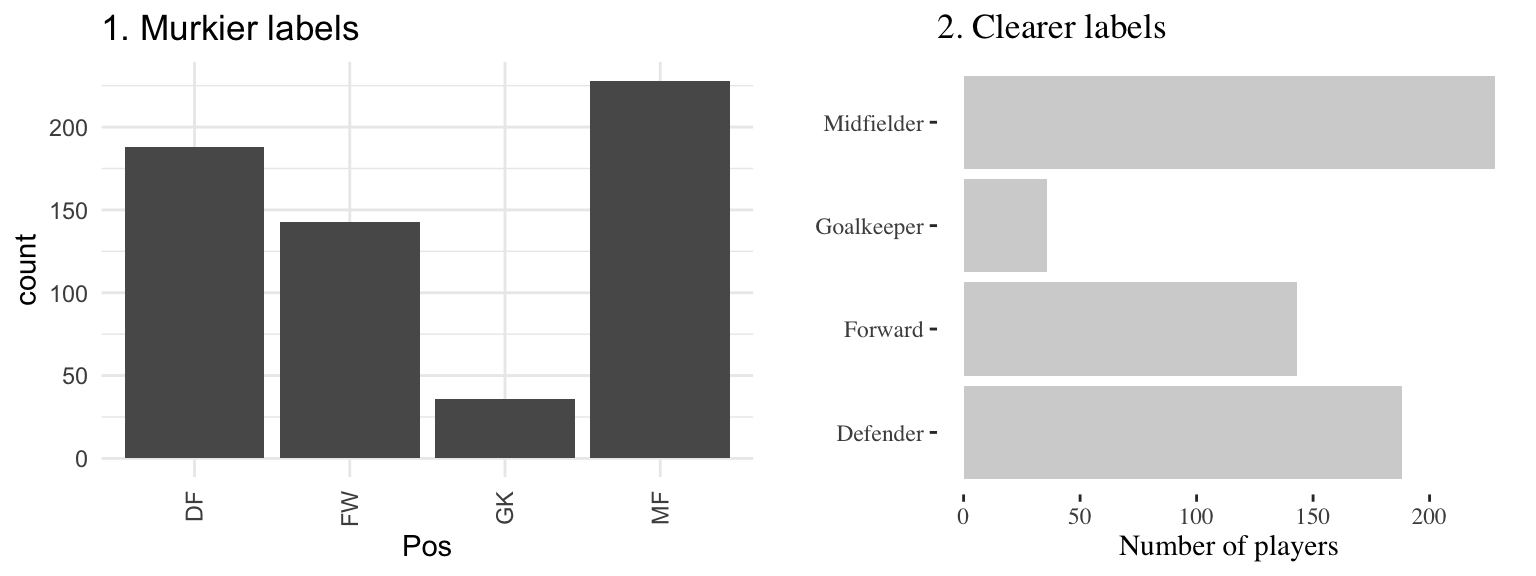Figure 4.23: The number of players in each position in the worldcup data from the faraway package. Both graphs show the same information, but the left graph has murkier labels, while the right graph has labels that are easier to read and interpret.

There are a few strategies you can use to make labels clearer when plotting with `ggplot2`:

• You can use the `xlab` and `ylab` functions to customize the axis labels on a ggplot object, rather than using the column names in the original data. You can use the `name` parameter of the `scale` family of functions (e.g., `scale_x_continuous`) to relabel x- and y-axes— these functions also give you the power to make other changes to the x- and y-axes (e.g., changing break points for the axis ticks). However, if you only need to change axis labels, `xlab` and `ylab` are often quicker.
• Use tidyverse functions to clean your data before plotting it. This is particularly useful if you need to change the labels of categorical data. You can pipe directly from tidyverse data cleaning into a ggplot call (see the example code below).
• Include units of measurement in axis titles when relevant. If units are dollars or percent, check out the `scales` package, which allows you to add labels directly to axis elements by including arguments like `labels = percent` in `scale` elements. See the helpfile for `scale_x_continuous` for some examples.
• If the x-variable requires longer labels, as is often the case with categorical data (for example, player positions Figure 4.23), consider flipping the coordinates, rather than abbreviating or rotating the labels. You can use `coord_flip` to do this.

For example, here is the code used to generate the plots similar to those in Figure 4.23 (we first create a version of the `worldcup` data with worse column names and factor labels to show how to improve these when creating a ggplot object):

``````library(forcats)
# Create a messier example version of the data
wc_example_data <- worldcup %>%
dplyr::rename(Pos = Position) %>%
mutate(Pos = fct_recode(Pos,
"DC" = "Defender",
"FW" = "Forward",
"GK" = "Goalkeeper",
"MF" = "Midfielder"))

wc_example_data %>%
ggplot(aes(x = Pos)) +
geom_bar()

wc_example_data %>%
mutate(Pos = fct_recode(Pos,
"Defender" = "DC",
"Forward" = "FW",
"Goalkeeper" = "GK",
"Midfielder" = "MF")) %>%
ggplot(aes(x = Pos)) +
geom_bar(fill = "lightgray") +
xlab("") +
ylab("Number of players") +
coord_flip() +
theme_tufte()``````

I> In this code example, we’ve used the `fct_recode` function from the `forcats` package to both create the messier example data and also to clean up category names for the second plot. The `forcats` package has a number of useful functions for working with factors in R.

W> In R, once you load a library, you do not specify that library when calling it’s function (e.g., once you’ve loaded `dplyr`, you can call `rename`). Usually, R does a good job of finding the right function under this system. However, if you have several packages loaded that have functions with the same name, you can run into problems. As you add on packages for plotting and mapping, you may find that some of your data cleaning code suddenly doesn’t work. If this happens, it may be that you’ve added code that loads the `plyr` package, which has several functions with the same name as `dplyr` functions. If this happens to you, try using the package::function notation to clarify that you want to use the `dplyr` function. You can see an example of this in the above code, where we’ve specified `dplyr::rename` when creating the messier example dataset.

#### 4.2.1.4 Highlighting

Guideline 4: Highlight interesting aspects.

Consider adding elements to highlight noteworthy elements of the data. For example, in the graph on the right of Figure 4.27, the days of the heat wave (based on temperature measurements) have been highlighted over the mortality time series by using a thick red line.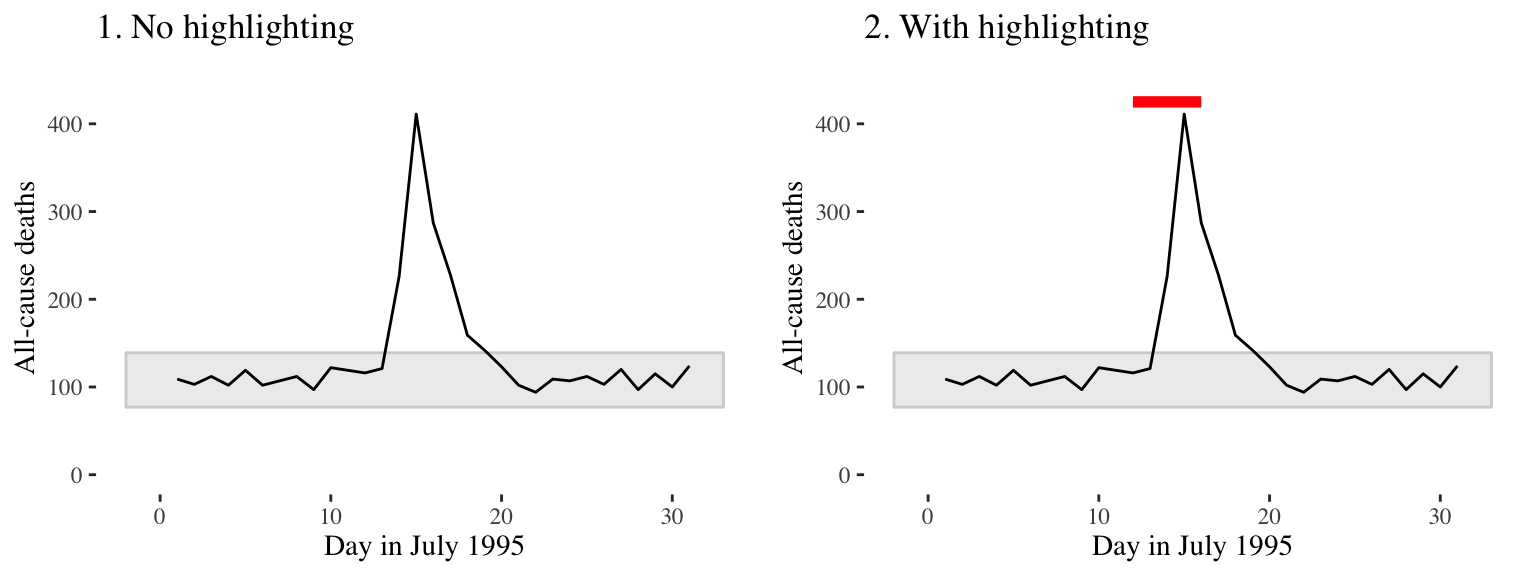Figure 4.27: Mortality in Chicago, July 1995. In the plot on the right, a thick red line has been added to show the dates of a heat wave.

In Figure 4.28, the names of the players with the most shots and passes have been added to highlight these unusual points.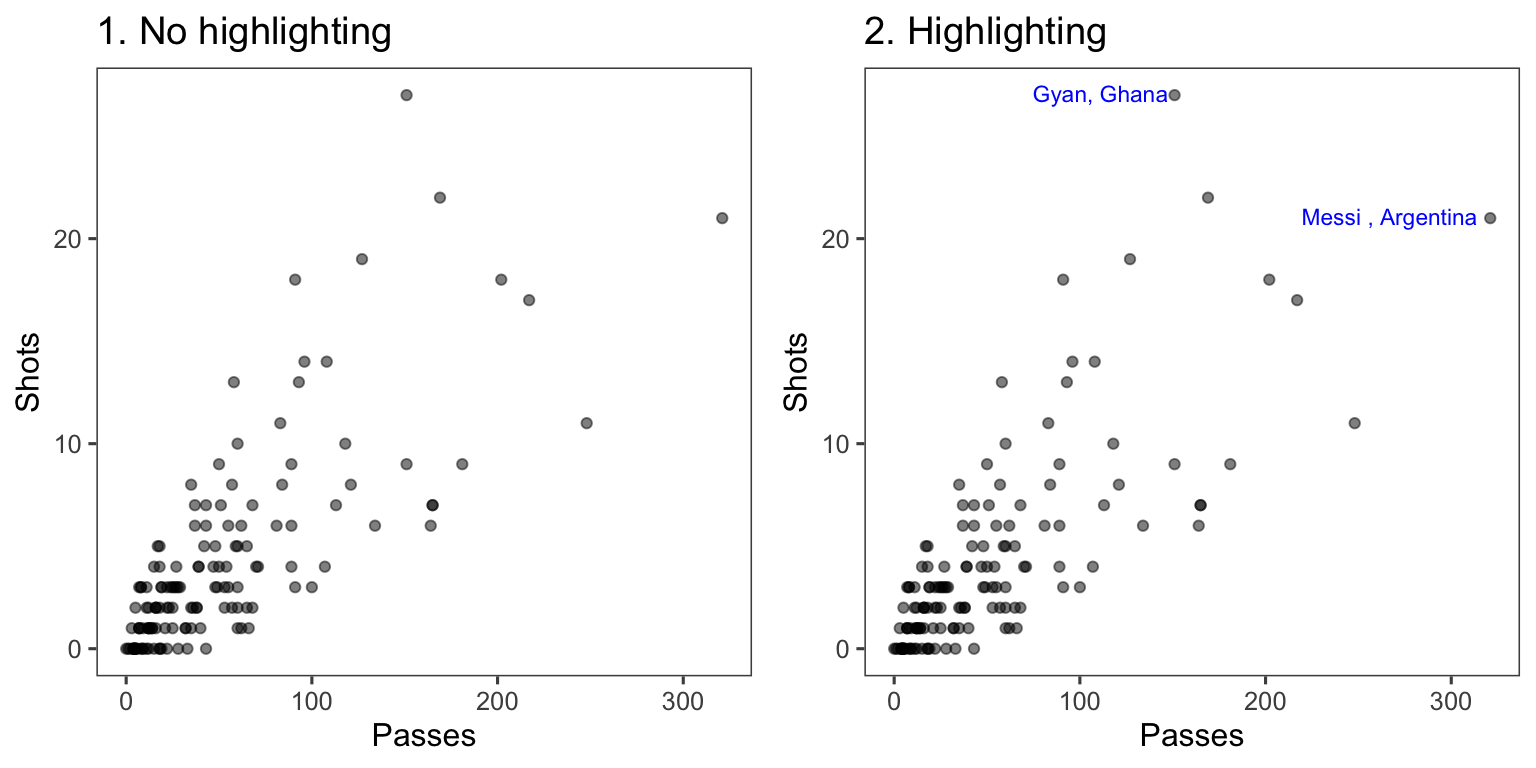Figure 4.28: Passes versus shots for World Cup 2010 players. In the plot on the right, notable players have been highlighted.

You can add highlighting elements using geoms like `geom_text` and `geom_line`. Often, you will need to use a different dataframe for this highlighting geom. For example, you may want to create a subset of the original dataframe with notable points to which you want to add text labels. You can specify a new dataframe for a geom using the `data` parameter in the function that adds that geom. For example, to create the right plot in Figure 4.28, we first created a subset dataframe with only the players with the most shots and passes (when creating this subset, we also included some code to create the text label we want to use in the plot):

``````noteworthy_players <- worldcup %>%
filter(Shots == max(Shots) | Passes == max(Passes)) %>%
mutate(point_label = paste0(Team, Position, sep = ", "))
noteworthy_players
Team   Position Time Shots Passes Tackles Saves       point_label
1 Ghana    Forward  501    27    151       1     0    GhanaForward,
2 Spain Midfielder  515     4    563       6     0 SpainMidfielder, ``````

Now you can create a ggplot object based on the `worldcup` data, add a point geom to create the scatterplot with all data, and then add the text geom with the data from noteworthy players to add labels for those players:

``````ggplot(worldcup, aes(x = Passes, y = Shots)) +
geom_point(alpha = 0.5) +
geom_text(data = noteworthy_players, aes(label = point_label),
vjust = "inward", hjust = "inward", color = "blue") +
theme_few()``````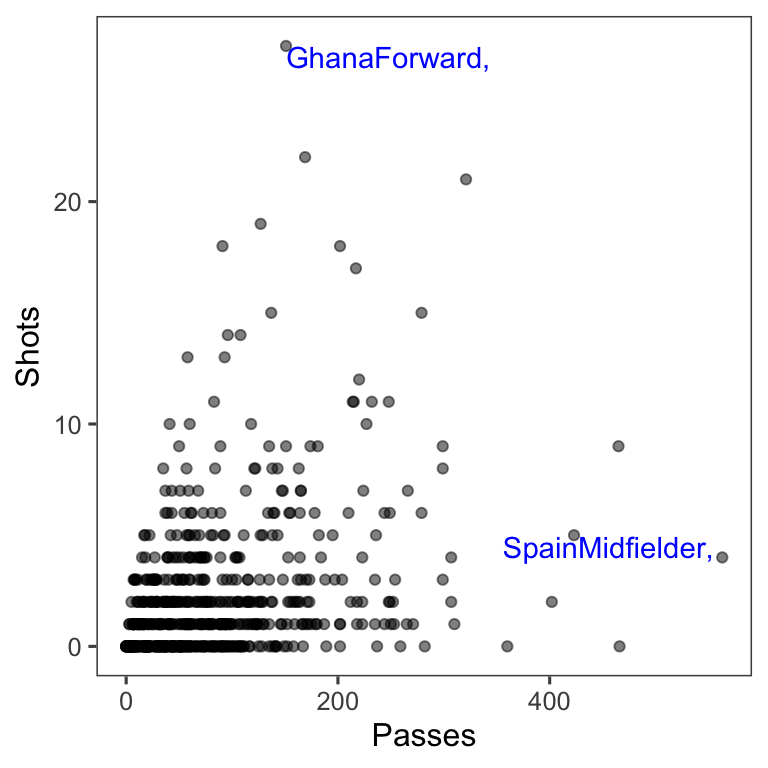#### 4.2.1.5 Small multiples

Guideline 5: When possible, use small multiples.

Small multiples are graphs that use many small plots to show different subsets of the data. Typically in small multiples, all plots use the same ranges for the x- and y-axes. This makes it easier to compare across plots, and it also allows you to save room by limiting axis annotation. In `ggplot2`, you can use faceting to creates small multiples.

For example, the `worldcup` dataset used in earlier examples includes each player’s position. If you want to explore a relationship (e.g., time played vs. shots on goal), you could try using color:

``````data(worldcup)
worldcup %>%
ggplot(aes(x = Time, y = Shots, color = Position)) +
geom_point() ``````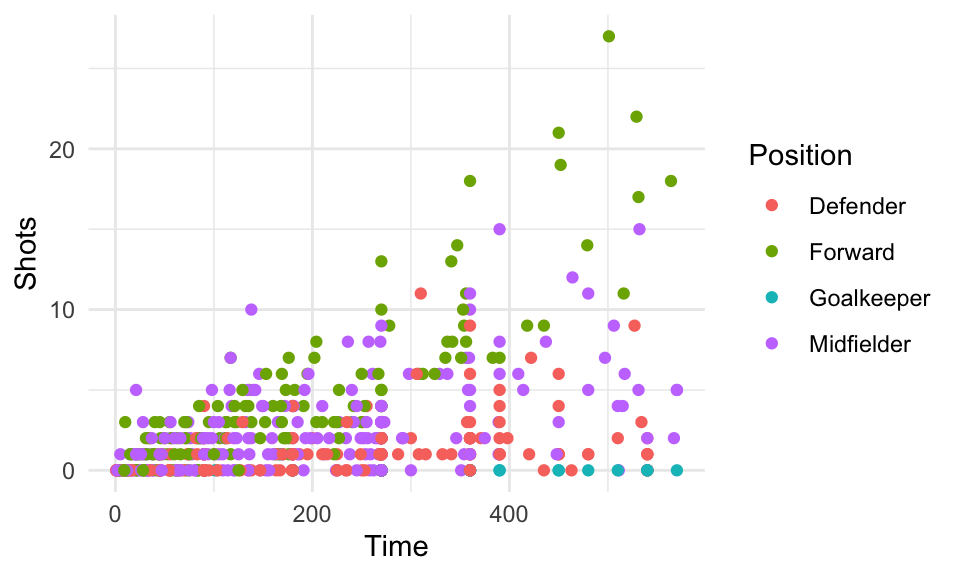Figure 4.29: Shots vs. Time by Position

However, often it’s clearer to see relationships if you use faceting instead to create a small separate plot for each position. You can do this with either the `facet_grid` function or the `facet_wrap` function:

``````worldcup %>%
ggplot(aes(x = Time, y = Shots)) +
geom_point() +
facet_grid(. ~ Position) ``````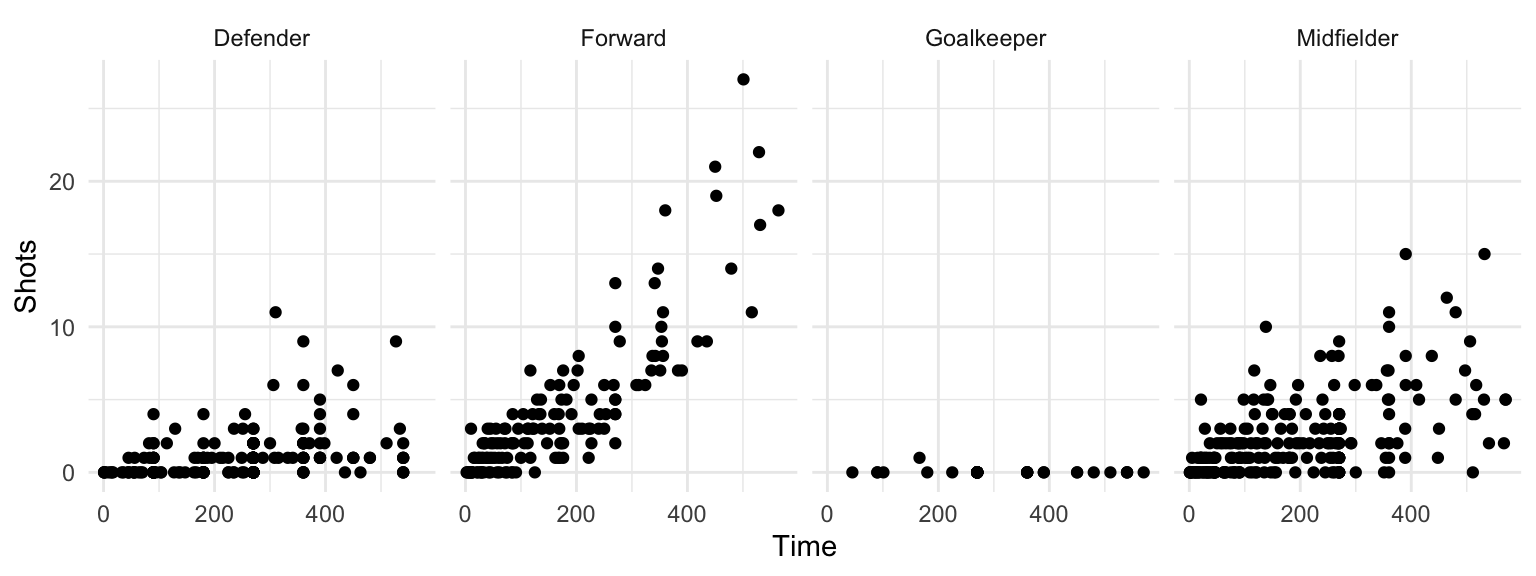Figure 4.30: Small multiples with facet_grid

The `facet_grid` and `facet_wrap` functions differ in whether the small graphs are placed with one faceting variable per dimension (`facet_grid`) or whether the plots are wrapped across several rows (`facet_wrap`).

The `facet_grid` function can facet by one or two variables. One will be shown by rows, and one by columns:

``````## Generic code
facet_grid([factor for rows] ~ [factor for columns])``````

The `facet_wrap()` function can facet by one or more variables, and it “wraps” the small graphs, so they don’t all have to be in one row or column:

``````## Generic code
facet_wrap(~ [formula with factor(s) for faceting],
ncol = [number of columns])``````

For example, if you wanted to show relationships for the final two teams in World Cup 2010 (Spain and Holland) and facet by both position and team, you could run:

``````worldcup %>%
filter(Team %in% c("Spain", "Netherlands")) %>%
ggplot(aes(x = Time, y = Shots)) +
geom_point() +
facet_grid(Team ~ Position) ``````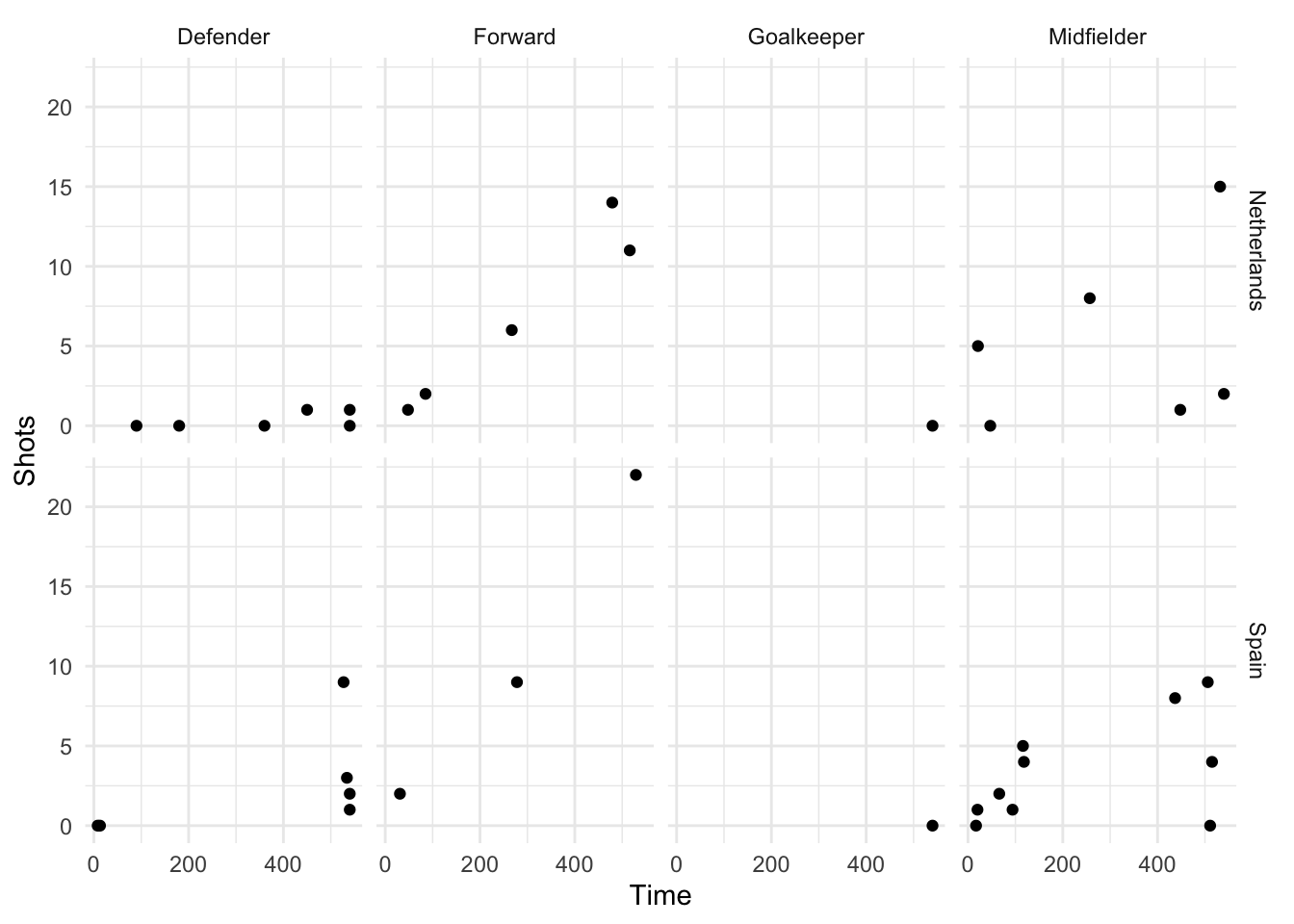Figure 4.31: Faceting by Position and Team

With `facet_wrap`, you can specify how many columns you want to use, which makes it useful if you want to facet across a variable with a lot of variables. For example, there are 32 teams in the World Cup. You can create a faceted graph of time played versus shots taken by team by running:

``````worldcup %>%
ggplot(aes(x = Time, y = Shots)) +
geom_point(alpha = 0.25) +
facet_wrap(~ Team, ncol = 6) ``````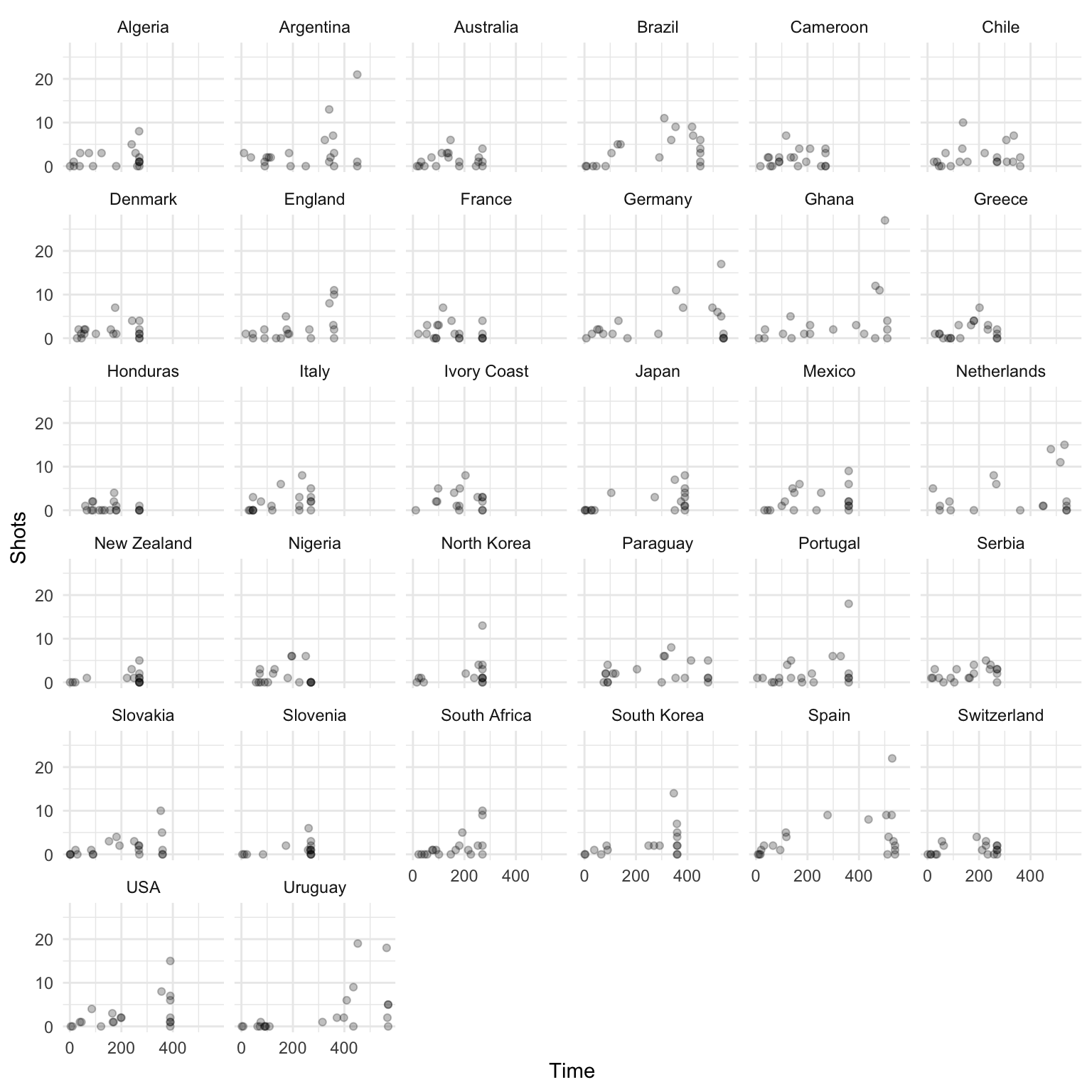Figure 4.32: Using facet_wrap

Often, when you facet a plot, you’ll want to re-name your factors levels or re-order them. For this, you’ll need to use the `factor()` function on the original vector, or use some of the tools from the `forcats` package. For example, to rename the `sex` factor levels from “1” and “2” to “Male” and “Female,” you can run:

``````nepali <- nepali %>%
mutate(sex = factor(sex, levels = c(1, 2),
labels = c("Male", "Female")))``````

Notice that the labels for the two graphs have now changed:

``````ggplot(nepali, aes(ht, wt)) +
geom_point() +
facet_grid(. ~ sex)``````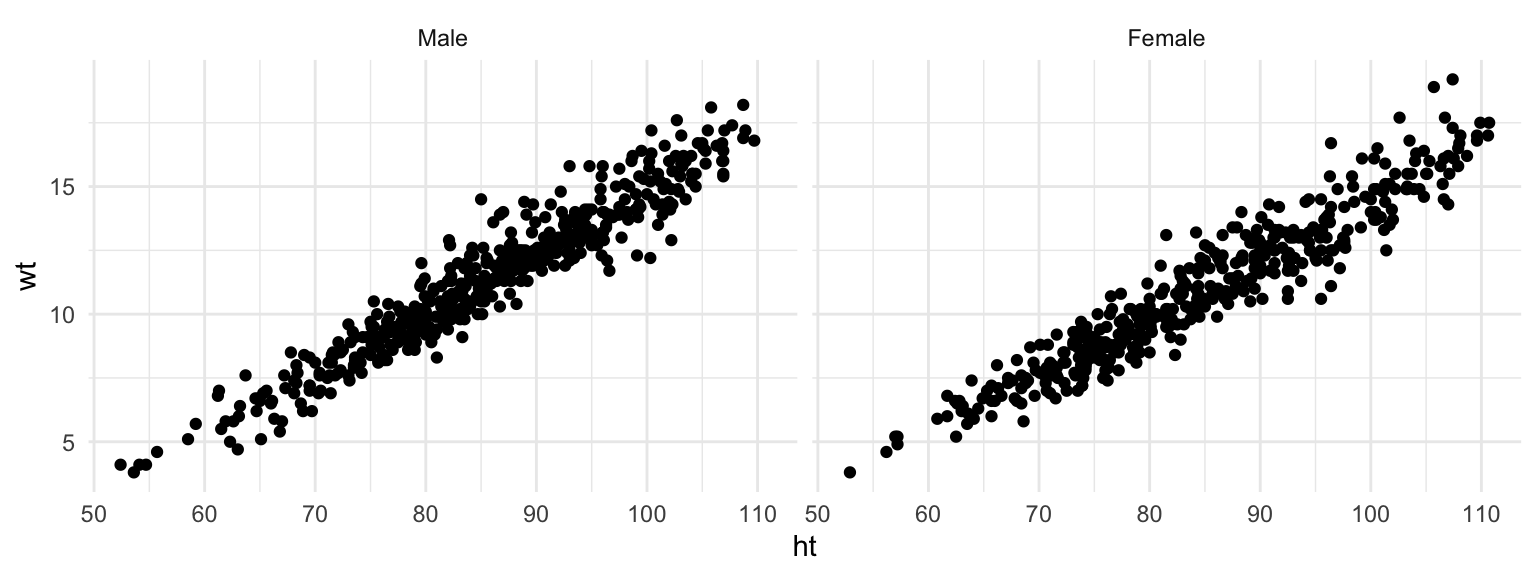Figure 4.33: Facets with labeled factor

To re-order the factor and show the plot for “Female” first, you can use `factor` to change the order of the levels:

``````nepali <- nepali %>%
mutate(sex = factor(sex, levels = c("Female", "Male")))``````

Now notice that the order of the plots has changed:

``````ggplot(nepali, aes(ht, wt)) +
geom_point() +
facet_grid(. ~ sex)``````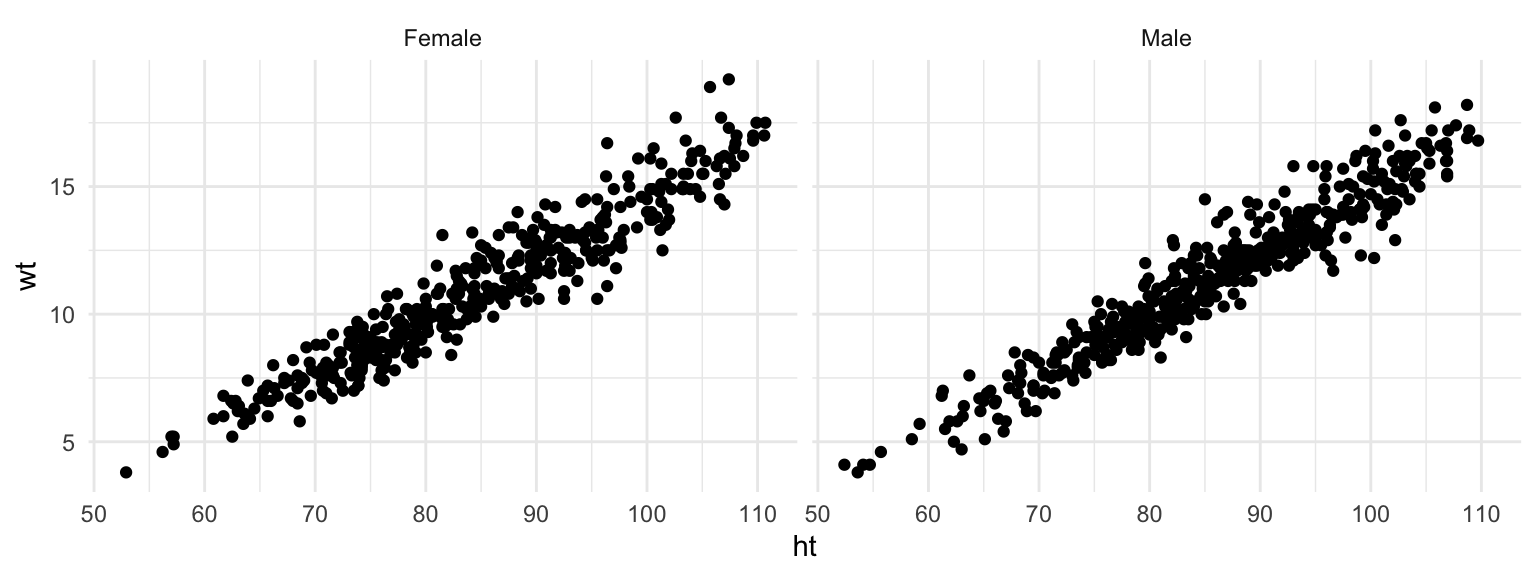Figure 4.34: Facets with re-labeled factor

#### 4.2.1.6 Order

Guideline 6: Make order meaningful.

Adding order to plots can help highlight interesting findings. Often, factor or categorical variables are ordered by something that is not interesting, like alphabetical order (Figure 4.35, left plot).

```summarise()` ungrouping output (override with `.groups` argument)``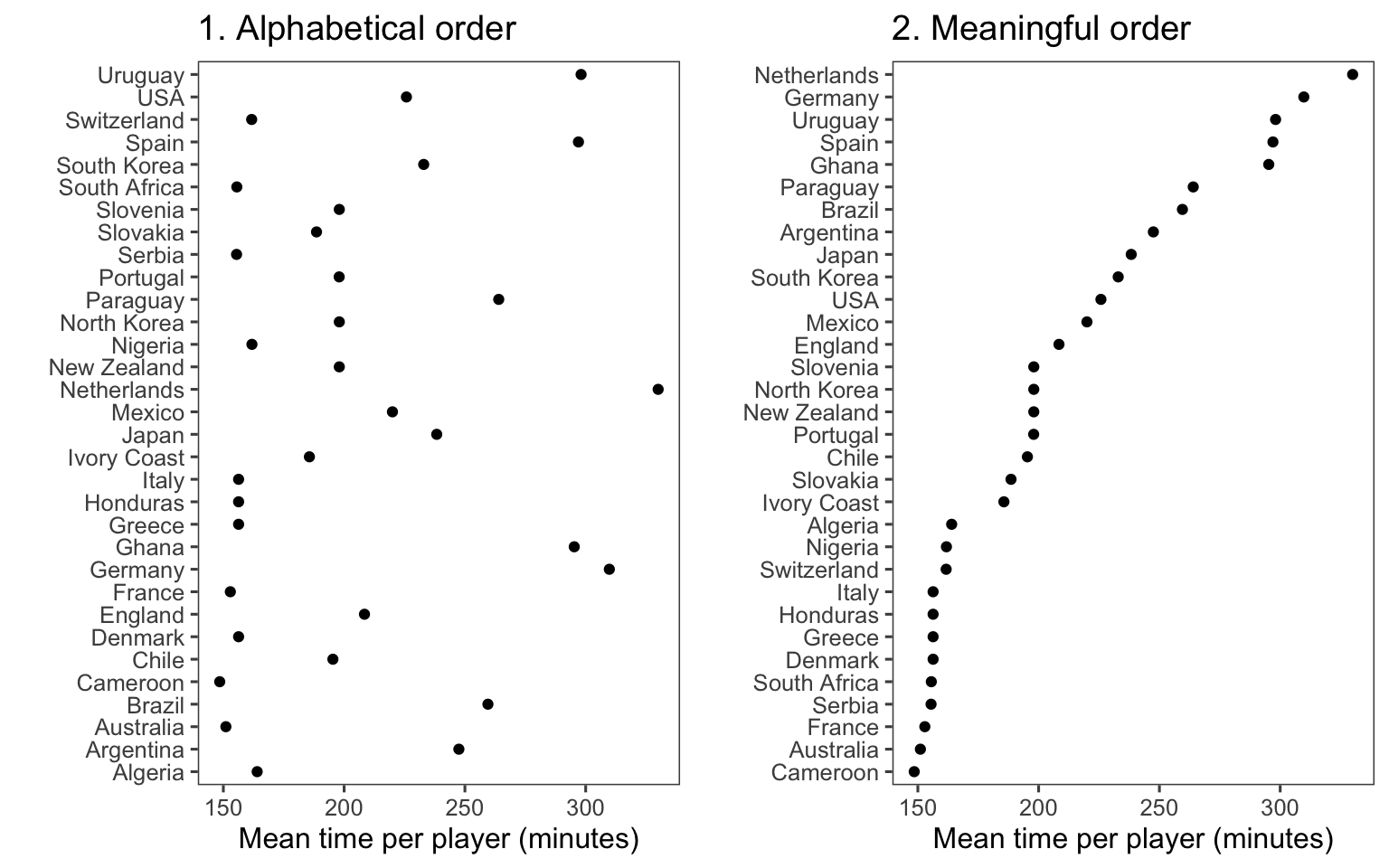Figure 4.35: Mean time per player in World Cup 2010 by team. The plot on the right has reordered teams to show patterns more clearly.

You can make the ranking of data clearer from a graph by using order to show rank (Figure 4.35, right). You can re-order factor variables in a graph by resetting the factor using the `factor` function and changing the order that levels are included in the `levels` parameter. For example, here is the code for the two plots in Figure 4.35:

``````## Left plot
worldcup %>%
group_by(Team) %>%
summarize(mean_time = mean(Time)) %>%
ggplot(aes(x = mean_time, y = Team)) +
geom_point() +
theme_few() +
xlab("Mean time per player (minutes)") + ylab("")

## Right plot
worldcup %>%
group_by(Team) %>%
summarize(mean_time = mean(Time)) %>%
arrange(mean_time) %>%                         # re-order and re-set
mutate(Team = factor(Team, levels = Team)) %>% # factor levels before plotting
ggplot(aes(x = mean_time, y = Team)) +
geom_point() +
theme_few() +
xlab("Mean time per player (minutes)") + ylab("") ``````

As another example, you can customize the faceted plot created in the previous subsection to order these plots from least to most average shots for a position using the following code. This example also has some added code to highlight the top players in each position in terms of shots on goal, as well as customizing colors and the theme.

``````worldcup %>%
select(Position, Time, Shots) %>%
group_by(Position) %>%
mutate(ave_shots = mean(Shots),
most_shots = Shots == max(Shots)) %>%
ungroup() %>%
arrange(ave_shots) %>%
mutate(Position = factor(Position, levels = unique(Position))) %>%
ggplot(aes(x = Time, y = Shots, color = most_shots)) +
geom_point(alpha = 0.5) +
scale_color_manual(values = c("TRUE" = "red", "FALSE" = "black"),
guide = FALSE) +
facet_grid(. ~ Position) +
theme_few()``````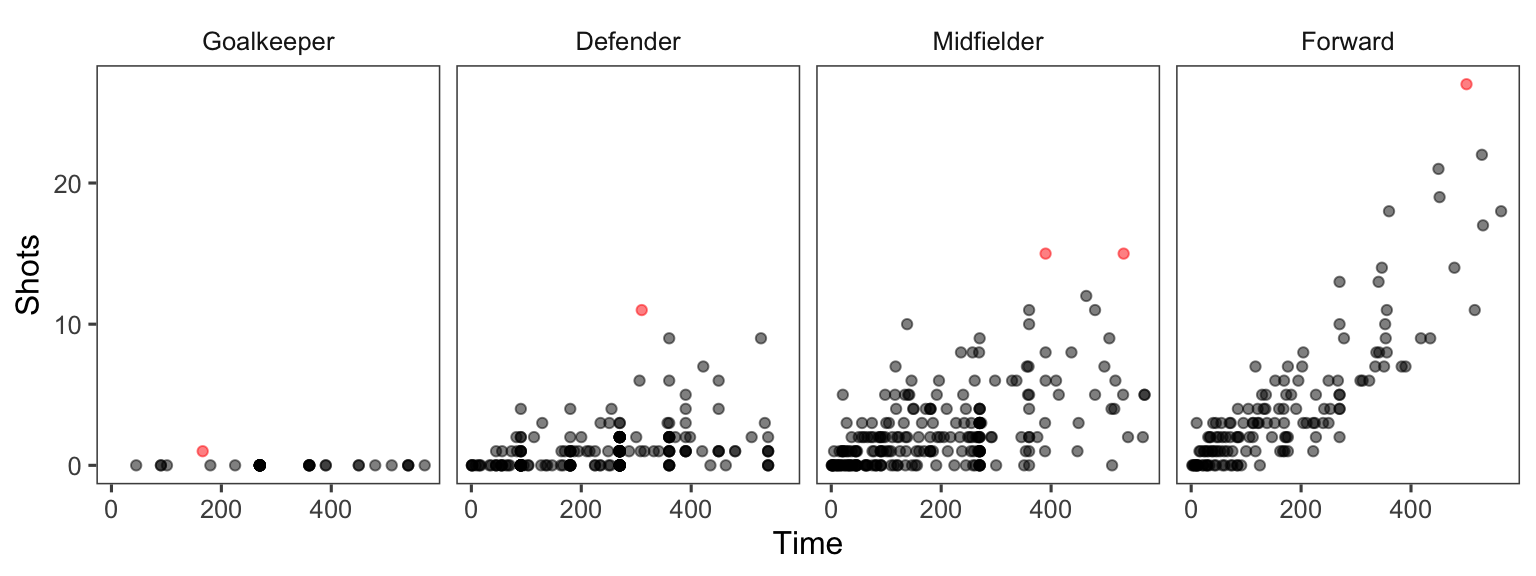Figure 4.36: More customization in faceting

As another example of ordering, suppose you wanted to show how playing times were distributed among players from each team for the World Cup data, with teams ordered by the average time for all their players. You can link up `dplyr` tools with `ggplot` to do this by using `group_by` to group the data by team, `mutate` to average player time within each team, `arrange` to order teams by that average player time, and `mutate` to reset the factor levels of the `Team` variable, using this new order, before plotting with `ggplot`:

``````worldcup %>%
dplyr::select(Team, Time) %>%
dplyr::group_by(Team) %>%
dplyr::mutate(ave_time = mean(Time),
min_time = min(Time),
max_time = max(Time)) %>%
dplyr::arrange(ave_time) %>%
dplyr::ungroup() %>%
dplyr::mutate(Team = factor(Team, levels = unique(Team))) %>%
ggplot(aes(x = Time, y = Team)) +
geom_segment(aes(x = min_time, xend = max_time, yend = Team),
alpha = 0.5, color = "gray") +
geom_point(alpha = 0.5) +
geom_point(aes(x = ave_time), size = 2, color = "red", alpha = 0.5) +
theme_minimal() +
ylab("")``````